Space, shape and measurement: Calculate perimeter, surface area and volume in two- and three-dimensional geometrical shapes

# Unit 1: Properties of polygons

Natashia Bearam-Edmunds### Unit outcomes: Unit 1: Properties of polygons

By the end of this unit you will be able to:

• Name the different types of polygons.
• Calculate the perimeter and area of the following:
• Square
• Rectangle
• Circle
• Triangle
• Parallelogram
• Trapezium
• Hexagon.
• Investigate the effect on the perimeter and surface area, where one or more dimensions are multiplied by a constant factor k.

## What you should know

Before you start this unit, make sure you can:

Solve algebraic equations. Go over Mathematics Level 2 Subject outcome 2.2 for more on algebraic equations.

## Introduction

Some common shapes that you already know are triangles, rectangles, hexagons and squares. The name we give to these types of shapes, formed with straight lines and that can be drawn on a flat (two-dimensional) surface is polygons. A circle is another common shape but it is not a polygon. Why do you think that is?

Circles and shapes with curves are not polygons. A polygon, by definition, is made up of straight lines. Four-sided polygons are called quadrilaterals. The quadrilaterals that you should be familiar with are parallelograms, rectangles, squares, and trapeziums.

## Types of polygons

Polygons are named according to the number of sides they have. They can have three or more sides. The names are based on the prefixes of Greek numbers. In fact, you will find references to Greek numerical prefixes in many everyday objects and this can help you remember the number of sides a polygon has. For example, a decade is $\scriptsize 10$ years and a decagon has $\scriptsize 10$ sides; an octopus has eight legs and an octagon has eight sides. Can you think of other examples that use Greek numerical prefixes?

There are two types of polygons, regular polygons and irregular polygons. A regular polygon has sides of equal length with equal angles between each side. In an irregular polygon, the sides have different lengths and the angles between the sides are unequal.

Figure 1 shows the different types of polygons that you may come across and examples of shapes that are not polygons.

### Note

When you have an internet connection watch the video called “Introduction to polygons” for a quick review of the different types of shapes.

Introduction to polygons (Duration: 2:24)Polygons (and other flat shapes) make up the base and sides (or faces) of many three-dimensional (3-D) shapes. In order to work out the volume and surface area of those objects we first need to know how to calculate the area and perimeter of two-dimensional (2-D) shapes.

## Calculating the surface area of polygons

You may have already calculated the surface area of objects without realising it. For example, when you cover a workbook or wrap a present you can calculate how much paper is needed to wrap the item so that you do not cut too little or too much paper. You need to understand shapes when doing home improvement, gardening and even when planning a party.

In manufacturing, working out the surface area and volume of objects is especially important to keep costs down and reduce wastage of material. We discuss volume and surface area in Units 2 and 3 of this subject outcome, but for now, we need to focus on the basics of area and perimeter.

Area is the size of the surface of a 2-D shape. It is the number of square units that fit onto a shape.

Perimeter is the total distance around any closed 2-D shape. The word comes from the Greek word peri (around) and meter (measure).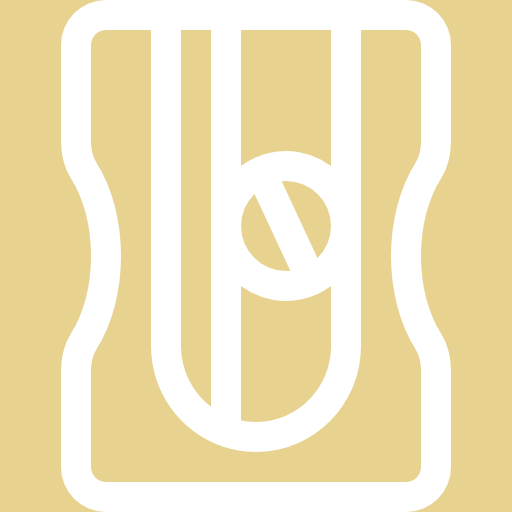### Activity 1.1: Find the area and perimeter of shapes

Time required: 15 minutes

What you need:

• a pen and paper

What to do:

Use the diagram below to answer the questions that follow.1. What is the area of A?
2. Approximately how much surface does B cover? How did you work it out?
3. Is there a difference between finding ‘the amount of surface covered’ and finding the ‘area’?
4. If each square on the graph paper is $\scriptsize 1\text{ cm}$ long and $\scriptsize 1\text{ cm}$ wide, what is the unit of measurement for the area of shapes A and B?
5. What is the perimeter of A?
6. What is the approximate perimeter of B?
7. What is the unit of measurement for the perimeter of shapes A and B?

What did you find?

1. The area of A is $\scriptsize 15$ square units.
2. B covers about $\scriptsize 6.5$ square units. We can count $\scriptsize 5$ full squares and two half squares, which makes a full square and then there is one more half square to add on.
3. Finding ‘the surface covered’ and finding the ‘area’ mean the same thing. It is helpful to think of ‘the surface covered’ when finding areas of faces, or sides, of geometric objects.
4. If each square on the graph paper is $\scriptsize 1\text{ cm}$ long and $\scriptsize 1\text{ cm}$ wide then the area of A and B will be in square centimetres or $\scriptsize \text{c}{{\text{m}}^{2}}$.
5. The perimeter of A is $\scriptsize 16$ units.
6. The approximate perimeter of B is $\scriptsize 13$ units.
7. The perimeter of A and B will be in centimetres or $\scriptsize \text{cm}$.

Counting squares is not the best or most accurate way to find the area of a shape, especially when edges don’t lie exactly on the square unit measures we are using, and this method can take a lot of time. So, mathematicians came up with formulae that we can use to calculate the area of different shapes. See Table 1 for these formulae:

You will notice that the only shape that we have not included in the table of formulae is the circle. This is because the circle deserves special mention. Before we look at the formula for area and perimeter of a circle, let’s revise the parts that make up a circle.

## Calculating the area of a circle

In a circle, all points on the edge are the same distance from the centre. The radius, $\scriptsize r$, of a circle is the length of a line drawn from the centre of the circle to any point on the circle. The diameter, $\scriptsize d$, is the length of a straight line drawn from one point on the circle through the centre of the circle to a point on the other side of the circle. The diameter is twice as long as the radius of a circle.

The perimeter of a circle is called the circumference. The really cool thing about circles is that when you divide the circumference by the diameter you get the same answer all the time! We call this number pi $\scriptsize (\pi )$. Pi is an irrational number which means that it cannot be written as a fraction and in decimal form, the decimal places go on forever and never repeat.

$\scriptsize \displaystyle \frac{{\text{circumference}}}{{\text{diameter}}}=\pi \approx 3.14159...$

So, when the diameter of a circle is one unit the circumference is about $\scriptsize 3.14$ units (here we have rounded pi to two decimal places).

The circumference of a circle is $\scriptsize \pi \times d$.

We have also seen that $\scriptsize d=2r$ so it makes sense that:

$\scriptsize \begin{array}{l}\text{circumference}&=\pi \times d\\&=\pi \times 2r\\&=2\pi r\end{array}$

The area of a circle is calculated using the formula $\scriptsize \text{Are}{{\text{a}}_{{\text{circle}}}}=\pi {{r}^{2}}$. For accuracy, always use the $\scriptsize \pi$ button on your calculator to calculate the area of a circle unless the question specifically states otherwise.### Example 1.1

Find the area of each of the polygons below:

1. .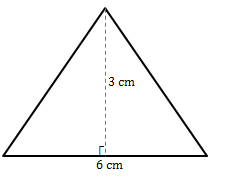2. .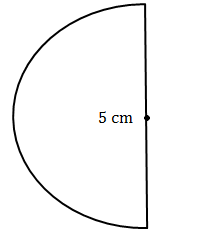3. (leave answer in surd form)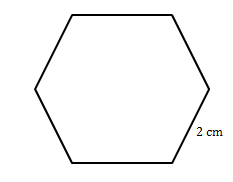Solutions

1. We are given the base and the perpendicular height of the triangle so we can substitute straight into the formula for area of a triangle.
$\scriptsize \begin{array}{l}\text{Area}&=\displaystyle \frac{1}{2}bh\\&=\displaystyle \frac{1}{2}(6)(3)\\&=9\text{ c}{{\text{m}}^{\text{2}}}\end{array}$
2. Here we have a semicircle, which is half the area of a full circle so we divide the formula by two.
$\scriptsize \begin{array}{l}\text{Area}&=\text{ }\displaystyle \frac{{\pi {{r}^{2}}}}{2}\text{ remember to use half the length of the diameter for the radius}\\&=\displaystyle \frac{{\pi {{{(2.5)}}^{2}}}}{2}\text{ }\\&=9.82\text{ c}{{\text{m}}^{2}}\end{array}$
3. We are given the side length of a regular hexagon so we use the formula $\scriptsize \text{Area}=\displaystyle \frac{{3\sqrt{3}}}{2}{{s}^{2}}$.
$\scriptsize \begin{array}{l}\text{Area}&=\displaystyle \frac{{3\sqrt{3}}}{2}{{s}^{2}}\\&=\displaystyle \frac{{3\sqrt{3}}}{2}{{(2)}^{2}}\\&=6\sqrt{3}\text{ c}{{\text{m}}^{2}}\end{array}$

Now, try this exercise on your own.### Exercise 1.1

1. Find the area of the following parallelogram.2. The following diagram shows a square attached to an isosceles triangle. The sides of the square are $\scriptsize 2$ metres in length, while the perpendicular height of the triangle is $\scriptsize 3$ metres.
1. Calculate the area of the shape.
2. If the equal sides of the triangle measure $\scriptsize \sqrt{{10}}$ metres calculate the perimeter of the shape (correct to one decimal place).3. Find the area of the trapezium shown below in terms of $\scriptsize x$.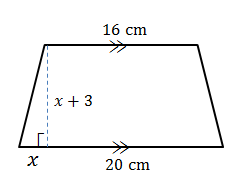The full solutions are at the end of the unit.

## Changing dimensions of a 2-D shape

Remember that a 2-D shape has two dimensions. As you have seen when we calculated areas, a rectangle has a length and a width (or breadth), a square has an equal length and a width, triangles and parallelograms have bases and heights, and so on. In simple terms, dimension is the size of the lengths that make up a shape.

Next, we investigate how changing one or both of the dimensions of a shape affects its perimeter and area.### Activity 1.2: Investigate the effect on area and perimeter when multiplying dimensions by a factor of k

Time required: 20 minutes

What you need:

• a pen and paper

What to do:

Each column below shows an original shape, the shape with one of its dimensions changed and the shape with both dimensions changed by the same value. Use the table to answer the questions that follow.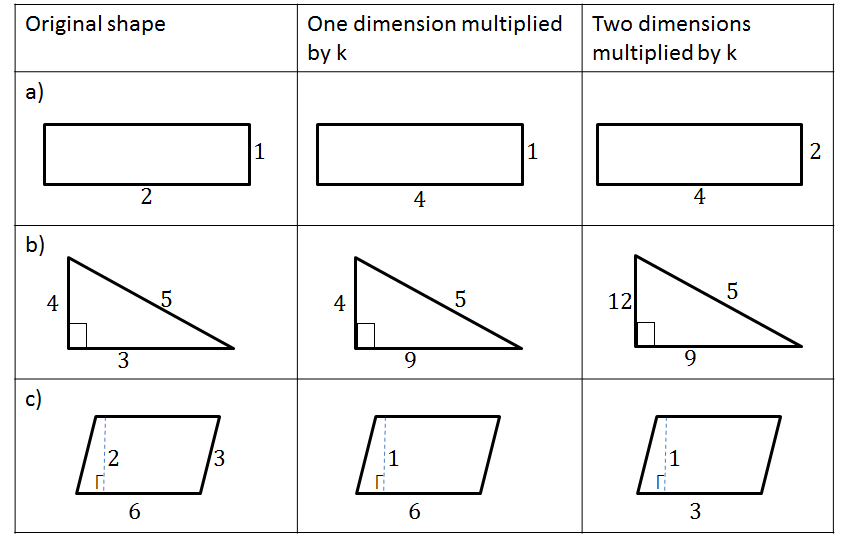1. State the value of $\scriptsize k$ in each case.
2. Find the area of the shapes in all three columns.
3. Compare the area of the original shape to the area when one dimension is changed. What can you conclude?
4. Compare the area of the original shape to the area when two dimensions are changed. What can you conclude?
5. Find the perimeter of the shapes in all three columns.
6. Compare the perimeter of the original shape to the perimeter when one dimension is changed. What can you conclude?
7. Compare the perimeter of the original shape to the perimeter when the two dimensions used to find the area of a shape are changed. What can you conclude?
8. What would happen to the perimeter if all the sides of a shape are multiplied by a factor of $\scriptsize k$? Find the perimeter in each case.

What did you find?

1. .
1. $\scriptsize k=2$
2. $\scriptsize k=3$
3. $\scriptsize k=\displaystyle \frac{1}{2}$
2. .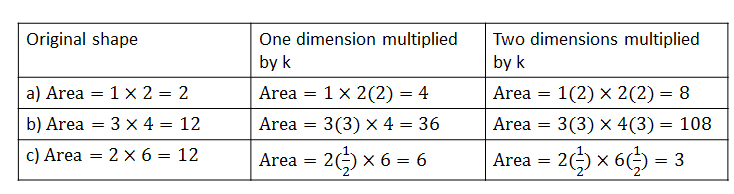3. When you multiply one dimension by $\scriptsize k$ then the new area is $\scriptsize k\times \text{original area}$.
4. When you multiply two dimensions by $\scriptsize k$ then the new area is $\scriptsize {{k}^{2}}\times \text{original area}$.
5. .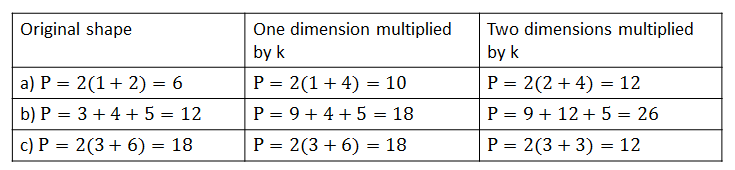6. There is no specific pattern that we see with perimeter when only one dimension is multiplied by a factor of $\scriptsize k$.
7. When the two dimensions used for the area are multiplied by a factor of $\scriptsize k$ there is no pattern observed in the perimeter.
8. If you multiply all the sides of a shape by a factor of $\scriptsize k$ the perimeter is $\scriptsize k\times \text{original perimeter}$.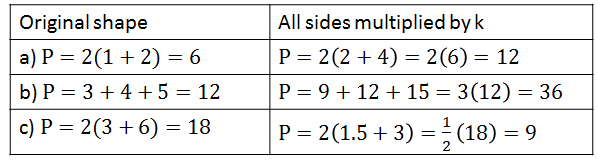We have seen that multiplying one or more dimensions by a factor of $\scriptsize k$ affects the area. If the dimensions that are changed are part of the area formula itself then we can conclude that:

1. When you multiply one dimension by $\scriptsize k$ then the new area is $\scriptsize k\times \text{original area}$.
2. When you multiply two dimensions by $\scriptsize k$ then the new area is $\scriptsize {{k}^{2}}\times \text{original area}$.

We have also found that when we multiply all the sides of a shape by a factor of $\scriptsize k$ then the perimeter is $\scriptsize k\times \text{original perimeter}$.### Example 1.2

1. A circular room has a radius of $\scriptsize x$.
1. Find the area of the room.
2. Find the circumference of the room.
3. If the radius is doubled find the new area and circumference of the room. Compare this to the original values.
2. The area of a rectangular table is $\scriptsize 2\text{ }{{\text{m}}^{2}}$. Without doing any further calculations find:
1. The area of the table if its length is doubled.
2. The area of the table if the length and width are doubled.

Solutions

1. .
1. .
$\scriptsize \begin{array}{l}\text{Area}&=\pi {{r}^{2}}\\&=\pi {{x}^{2}}\end{array}$
2. $\scriptsize Circumference=2\pi (x)$
3. The new radius will be $\scriptsize 2x$.
.
$\scriptsize \begin{array}{l}\text{Are}{{\text{a}}_{{\text{new}}}}&=\pi {{(2x)}^{2}}\\&=4\pi {{x}^{2}}\end{array}$
.
When you double the radius, the area increases by four times since the radius is squared in the area formula.

$\scriptsize \begin{array}{l}\text{Perimete}{{\text{r}}_{{\text{new}}}}&=2\pi (2x)\\&=4\pi x\end{array}$
When the radius is doubled, the circumference is doubled too.

The area of a circle is directly proportional to the square of the radius of the circle. This means that if the radius is increased by a factor of $\scriptsize k$, its area will be increased to $\scriptsize {{k}^{2}}$ times the original area.

The circumference is directly proportional to the size of the radius so if the radius of a circle is increased by a factor of $\scriptsize k$, its circumference will increase by a factor of $\scriptsize k$ too.

1. .
1. When you multiply one dimension by $\scriptsize k$ then the new area is $\scriptsize k\times \text{original area}$.
.
$\scriptsize \begin{array}{l}\text{Are}{{\text{a}}_{{\text{new}}}}&=2\times 2\text{ }{{\text{m}}^{2}}\\&=4\text{ }{{\text{m}}^{2}}\end{array}$
2. When you multiply two dimensions by $\scriptsize k$ then the new area is $\scriptsize {{k}^{2}}\times \text{original area}$.
.
$\scriptsize \begin{array}{l}\text{Are}{{\text{a}}_{{\text{new}}}}&={{(2)}^{2}}\times 2\text{ }{{\text{m}}^{2}}\\&=8\text{ }{{\text{m}}^{2}}\end{array}$

## Summary

In this unit you have learnt the following:

• How to identify different polygons.
• How to find the area of a square, rectangle, circle, triangle, parallelogram, trapezium and hexagon.
• How multiplying the dimensions by a scale factor of $\scriptsize k$ affects the area.
• How multiplying the dimensions by a scale factor of $\scriptsize k$ affects the perimeter.
• How multiplying all the sides by a scale factor of $\scriptsize k$ affects the perimeter.

# Unit 1: Assessment

#### Suggested time to complete: 20 minutes

1. The figure below shows the floor plan of a room. Find the area of the room.2. The shape below is that of a window, consisting of a rectangular section $\scriptsize \text{HJKL}$ and a semi-circular top section. $\scriptsize \text{HJ}=0.5\text{ m}$ and $\scriptsize \text{JK}=0.2\text{ m}$. Calculate the area and perimeter.3. A rectangular storeroom has length $\scriptsize 3x$ and width $\scriptsize 6y$. Write simplified expressions for the:
1. area of the storeroom.
2. perimeter of the storeroom.
3. If the dimensions of the storeroom are tripled, write down the new simplified expression for the area of the storeroom.
4. The following diagram shows a field in the shape of a trapezium. Liza will run in a straight line from $\scriptsize \text{V}$ to $\scriptsize \text{E}$ to $\scriptsize \text{L}$ to $\scriptsize \text{D}$ to train for a race.
Use the sketch to calculate the total distance she runs.
(HINT: Use Pythagoras)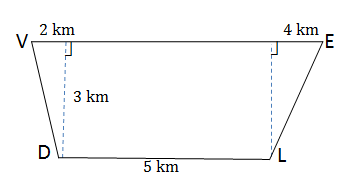The full solutions are at the end of the unit.

# Unit 1: Solutions

### Exercise 1.1

1. The opposite sides of a parallelogram (parm for short) are equal therefore, the base of the parm is $\scriptsize 10\text{ cm}$.
Use the formula $\scriptsize \text{Are}{{\text{a}}_{{parm}}}=b\times h$
$\scriptsize \begin{array}{l}\text{Are}{{\text{a}}_{{parm}}}&=10\text{ cm}\times 4\text{ cm}\\&=40\text{ c}{{\text{m}}^{2}}\end{array}$
2. The base of the triangle is a side of the square.
1. .
$\scriptsize \begin{array}{l}\text{Total area}&=\text{Area of square}+\text{Area of triangle}\\&={{l}^{2}}+\displaystyle \frac{1}{2}b\cdot h\\&=2\text{ }{{\text{m}}^{2}}+\displaystyle \frac{1}{2}(2\text{ m})\cdot (3\text{ m})\\&=2\text{ }{{\text{m}}^{2}}+3\text{ }{{\text{m}}^{2}}\\&=5\text{ }{{\text{m}}^{2}}\end{array}$
2. Be careful not to count the length that forms part of the boundary of the square and triangle.
.
$\scriptsize \begin{array}{l}\text{Perimeter}&=2\text{ m}+2\text{ m}+2\text{ m}+\sqrt{{10}}\text{ m}+\sqrt{{10}}\text{ m}\\&=6+2\sqrt{{10}}\\&=12.3\text{ m}\end{array}$
3. .
$\scriptsize \begin{array}{l}\text{Are}{{\text{a}}_{{\text{trapezium}}}}&=\displaystyle \frac{1}{2}\left( {\text{sum of parallel sides}} \right)\times h\\&=\displaystyle \frac{1}{2}\left[ {(x+20)+16} \right]\cdot (x+3)\\&=\displaystyle \frac{1}{2}(x+36)(x+3)\\&=\displaystyle \frac{1}{2}({{x}^{2}}+39x+108)\\&=\displaystyle \frac{1}{2}{{x}^{2}}+\displaystyle \frac{{39}}{2}x+54\end{array}$

Back to Exercise 1.1

### Assessment

1. We can calculate the area of the room by dividing the floor into two rectangles.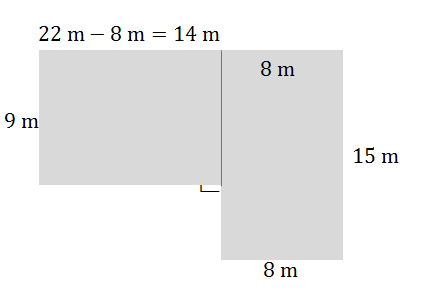$\scriptsize \begin{array}{l}\text{Area}&=(l\times b)+(l\times b)\\&=(14\times 9)+(15\times 8)\\&=126+120\\&=246\ {{\text{m}}^{\text{2}}}\end{array}$
2. .
$\scriptsize \begin{array}{l}\text{Area}&=\displaystyle \frac{{\pi {{r}^{2}}}}{2}+l\times b\\&=\left( \frac{{\pi {{{(0.25)}}^{2}}}}{2}} \right)+(0.2\times 0.5)\\&=0.2\ {{\text{m}}^{2}}\end{array$
3. .
1. .
$\scriptsize \begin{array}{l}\text{Area}&=3x\times 6y\\&=18xy\end{array}$
2. .
$\scriptsize \begin{array}{l}\text{Perimeter}&=2(3x+6y)\\&=6x+12y\end{array}$
3. .
$\scriptsize \begin{array}{l}\text{Are}{{\text{a}}_{{\text{new}}}}&=({{3}^{2}})18xy\\&=162xy\end{array}$
4. Calculate $\scriptsize \text{VD}$ and $\scriptsize \text{EL}$ by Pythagoras. The perpendicular distance between parallel lines is always equal, therefore the distance between $\scriptsize \text{VE}$ and $\scriptsize \text{DL}$ is $\scriptsize 3\text{ m}$.$\scriptsize \begin{array}{l}\text{V}{{\text{D}}^{2}}&={{(2)}^{2}}+{{(3)}^{2}}\text{ (Pythagoras)}\\&=4+9\\\therefore \text{VD}&=\sqrt{{13}}\text{ km}\end{array}$
$\scriptsize \begin{array}{l}\text{E}{{\text{L}}^{2}}&={{(4)}^{2}}+{{(3)}^{2}}\text{ (Pythagoras)}\\&=16+9\\\text{VD}&=\sqrt{{25}}\\&=5\text{ km}\end{array}$
$\scriptsize \begin{array}{l}\text{VD}+\text{VE}+\text{EL}+\text{LD}&=\sqrt{{13}}\text{ km }+2\text{ km}+5\text{ km}+4\text{ km}+5\text{ km}+5\text{ km}\\&=\text{ }\sqrt{{13}}\text{ km }+21\text{ km}\\&=24.6\text{ km}\end{array}$

Back to Unit 1: Assessment